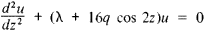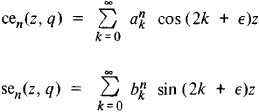# Mathieu Functions

## Mathieu functions

[ma′tyü ‚fəŋk·shənz]
(mathematics)
Any solution of the Mathieu equation which is periodic and an even or odd function.
McGraw-Hill Dictionary of Scientific & Technical Terms, 6E, Copyright © 2003 by The McGraw-Hill Companies, Inc.
The following article is from The Great Soviet Encyclopedia (1979). It might be outdated or ideologically biased.

## Mathieu Functions

special functions introduced by the French mathematician E. Mathieu in 1868 in the course of his work on the vibrations of an elliptical membrane. Mathieu functions are also used in the study of the propagation of electromagnetic waves in an elliptical cylinder and in the examination of tidal waves in a container having the shape of an elliptical cylinder. Even or odd functions that are periodic solutions of the linear second-order differential equation (Mathieu’s equation)are termed Mathieu functions. The condition that the solution of this equation be periodic determines a sequence of values for λ dependent on q. If q = 0, then λ = n2 (n = 1, 2, …), and the Mathieu functions in this case are cos nz and sin nz. For q ≠ 0, the Mathieu functions, denoted by cen(z, q) and sen(z, q), can be represented in the formwhere ank and bnk depend on q; ε = 0 for even n and ε = 1 for odd n.

### REFERENCES

Whittaker, E. T., and G. N. Watson.Kurs sovremennogo analiza, part 2, 2nd ed. Moscow, 1963. (Translated from English.)
McLachlan, N. W. Teoriia i prilozhentiia funktsii Mat’e. Moscow, 1953. (Translated from English.)
Mentioned in ?
References in periodicals archive ?
There are two books on functions which are special cases of the Heun Equation: Mathieusche Funktionen und Sphaeroidfunktionen mit anwendungen auf physikalische und technische Probleme by Joseph Meixner and Friedrich Wilhelm Schaefke, published by Springer Verlag in 1954  and a Dover reprint of a book first published in 1946, Theory and Applications of Mathieu Functions by N.
The wave equation for the scalar particle in the background of the Eguchi-Hanson metric  in four dimensions has hypergeometric functions as solutions , whereas the Nutku helicoid [27, 28] metric, the next higher one, gives us Mathieu functions , a member of the Heun function set, if the method of separation of variables is used to get a solution.
In the next sections we will outline the one coupled mode approximation (OCMA), the Mathieu functions approximation, and the theory of finite periodic systems (TFPS).
In the next sections we will present the Mathieu functions approach and the theory of finite periodic systems and, with the purpose of comparing with the results shown in Figure 1, we will calculate the same physical quantities for a system similar to that considered here.
The results obtained will be compared with those obtained by using Kogelnik's coupled wave theory and a matrix method which gives exact solutions in terms of Mathieu functions. In Sections 3, 4, and 5, the general solution is particularized for the cases of a homogeneous dielectric slab (no index modulation), an index-matched dielectric grating, and a partially index-matched dielectric grating, respectively.
The matrix method gives exact results for the efficiency of the first diffracted order in terms of Mathieu functions. The parameters used in the simulations throughout the text, such as the refractive index, the refractive index modulation, or the thickness of the layer, were chosen to represent reflection diffraction gratings recorded in photopolymers [20-23].
The elements of the layer matrix which characterizes the reflection grating are calculated in terms of Mathieu functions. In addition the band structure for a general non-slanted reflection grating is obtained by using the layer matrix corresponding to one single period demonstrating that no stop bands exist and that the band edges coincide with the Bragg angles of Kogelnik's Theory.
Portugal, "Algebraic methods to compute Mathieu functions," J.
The solution of such an equation invariably leads to Mathieu functions [Alhargan 1996; Alhargan and Judah 1992; McLachlan 1947; Morse and Feshbach 1953; NBS 1967].
Many algorithms have been devised to compute Mathieu functions with various degrees of success [Alhargan 1996; Arscott and Shymansky 1978; Blanch 1966; Leeb 1979; McLachlan 1947; Morse and Feshbach 1953; NBS 1967; Rengarajan and Lewis 1980; Shirts 1993a; 1993b; Toyama and Shogan 1984; Wimp 1984].
This article presents the outline of a set of routines that enable the computation of all Mathieu functions of integer orders for large range of the order n and the parameter h.
The accuracy of the results can be checked using the Wronskians for Mathieu functions, as the algorithms for computing Mathieu functions are not based on the Wronskian of the functions.

Site: Follow: Share:
Open / Close